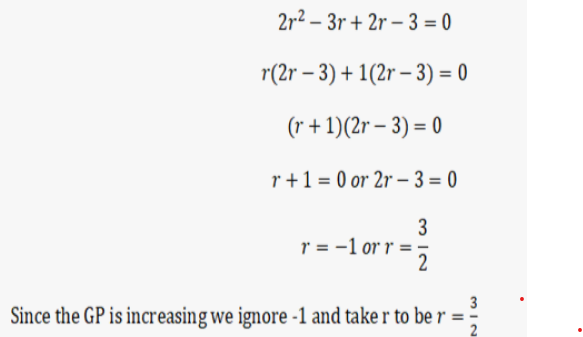+2546102301456

#### Mathematics Answering Techniques per Topic

- (Ratios and Proportions)

Q1.- (series and sequences)

Q2.- (series and sequences)

Q3.- (Three Dimensional Geometry (Maths Paper 2 2019 No. 11 (a) ))

Q4.- (RECIPROCALS)

Q5.- (Arithmetic progression (A.P))

Q6.- (BINOMIAL EXPANSIONS)

Q7.